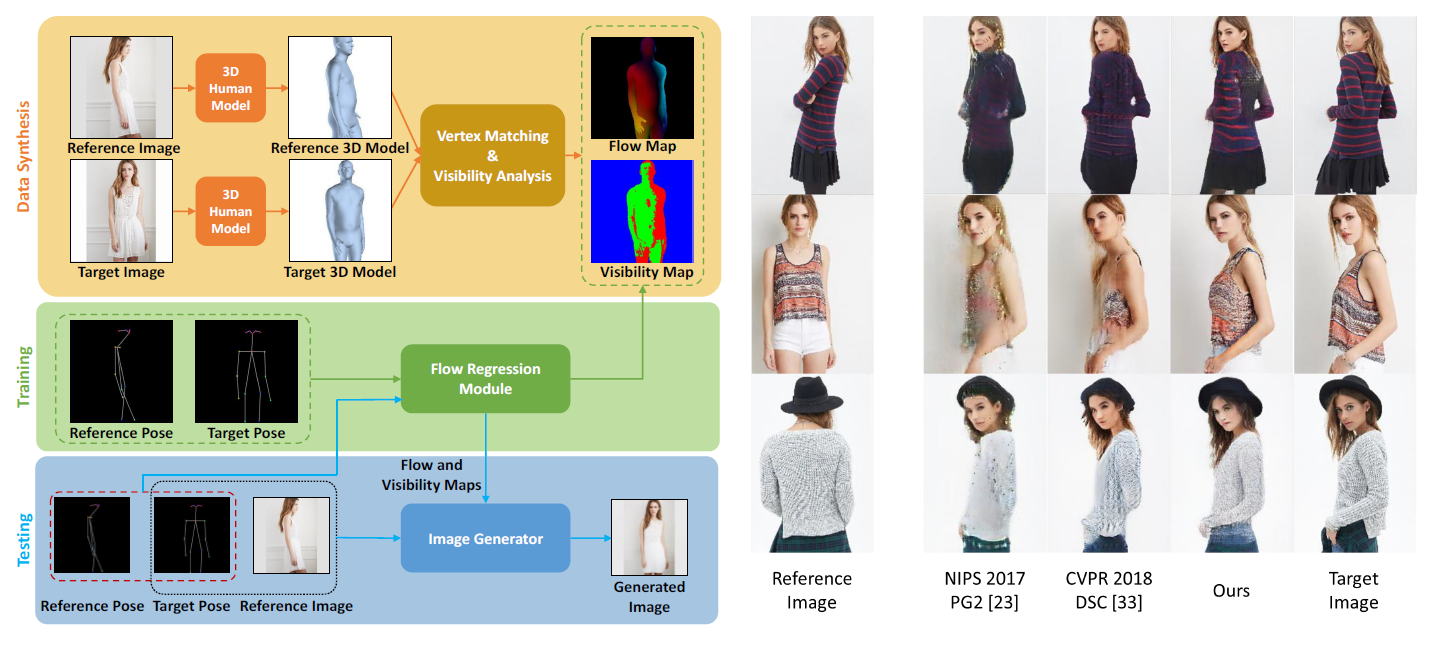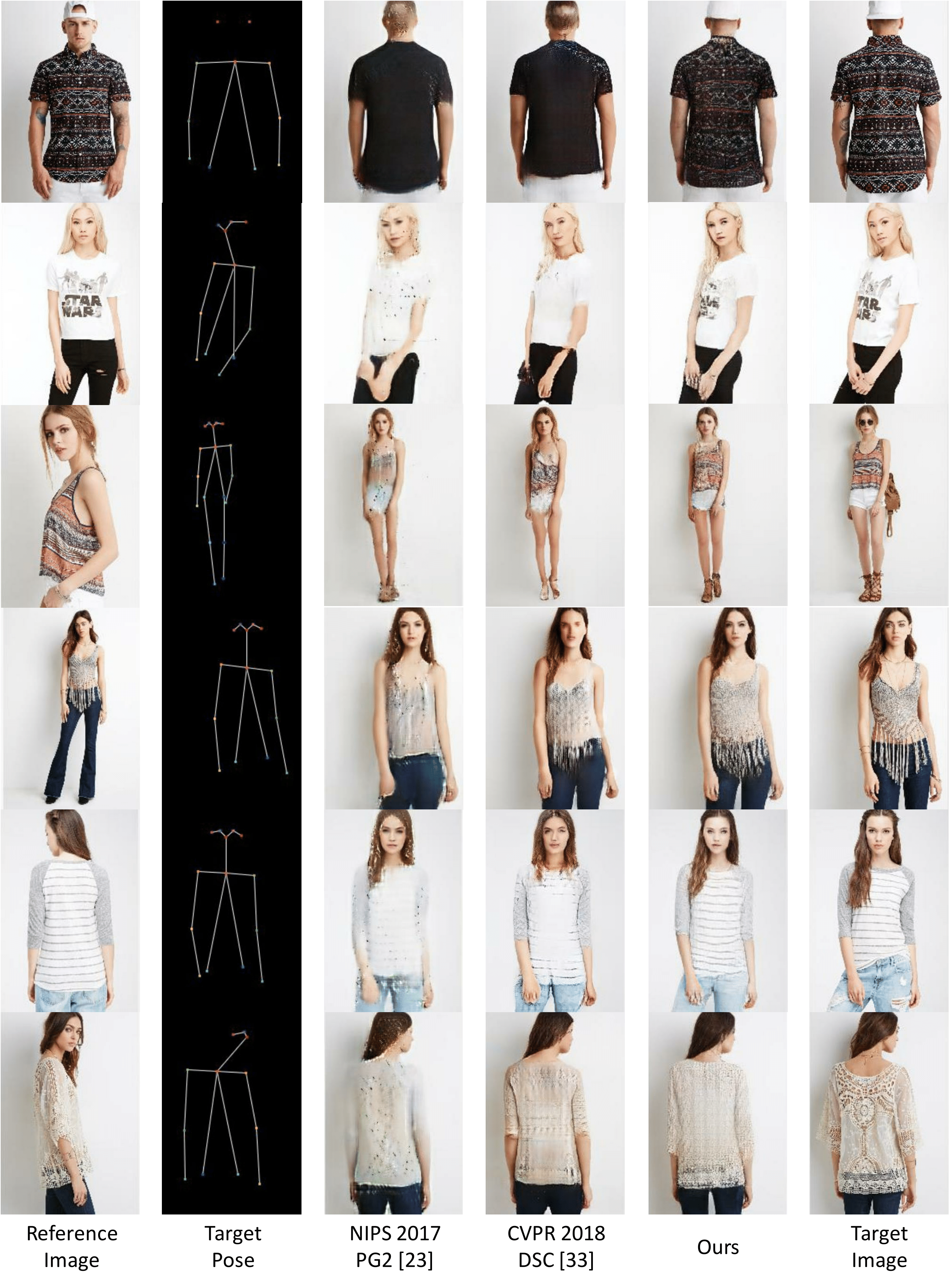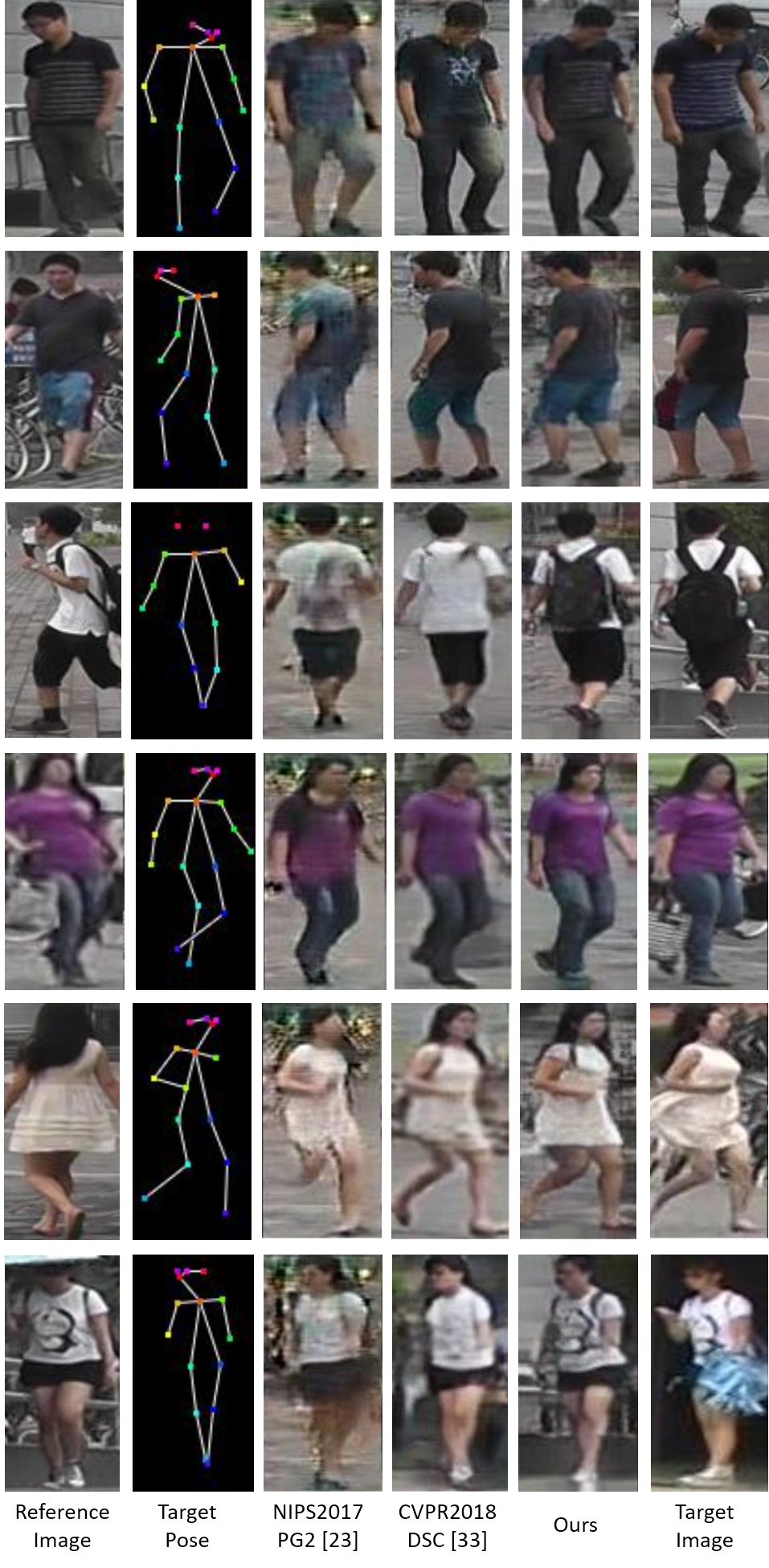# Dense Intrinsic Appearance Flow for Human Pose Transfer

Yining Li1, Chen Huang2 and Chen Change Loy3

1CUHK-SenseTime Joint Lab, The Chinese University of Hong Kong

2Robotics Institute, Carnegie Mellon University

3School of Computer Science and Engineering, Nanyang Technological University

IEEE Conference on Computer Vision and Pattern Recognition (CVPR) 2019

[Paper]     [Supplementary]     [Code]

## Abstract

We present a novel approach for the task of human pose transfer, which aims at synthesizing a new image of a person from an input image of that person and a target pose. We address the issues of limited correspondences identified between keypoints only and invisible pixels due to self-occlusion. Unlike existing methods, we propose to estimate dense and intrinsic 3D appearance flow to better guide the transfer of pixels between poses. In particular, we wish to generate the 3D flow from just the reference and target poses. Training a network for this purpose is non-trivial, especially when the annotations for 3D appearance flow are scarce by nature. We address this problem through a flow synthesis stage. This is achieved by fitting a 3D model to the given pose pair and project them back to the 2D plane to compute the dense appearance flow for training. The synthesized ground-truths are then used to train a feedforward network for efficient mapping from the input and target skeleton poses to the 3D appearance flow. With the appearance flow, we perform feature warping on the input image and generate a photorealistic image of the target pose. Extensive results on DeepFashion and Market-1501 datasets demonstrate the effectiveness of our approach over existing methods.The proposed human pose transfer method with dense intrinsic 3D appearance flow generates higher quality images in comparison to baselines. (Left) The core of our method is a flow regression module (the green box) that can transform the reference and target poses into a 3D appearance flow map and a visibility map.

## Intrinsic Appearance Flow

The proposed dense intrinsic appearance flow consists of two components, namely a flow map $$F$$ and a visibility map $$V$$ between image pair $$(x_1,x_2)$$ to jointly represent their pixel-wise correspondence in 3D space. Note $$F$$ and $$V$$ have the same spatial dimensions as the target image $$x_2$$. Assume that $$u_i'$$ and $$u_i$$ are the 2D coordinates in images $$x_1$$ and $$x_2$$ that are projected from the same 3D body point $$h_i$$, $$F$$ and $$V$$ can be defined as:

$$f_i=F(u_i)=u_i'-u_i,$$

$$v_i=V(u_i)=visibility(h_i,x_1),$$

where $$visibility(h_i,x_1)$$ is a function that indicates whether $$h_i$$ is invisible (due to self-occlusion or out of the image plane) in $$x_1$$. It outputs 3 discrete values (representing visible, invisible or background) which are color-coded in a visibility map $$V$$ (see an example in the figure).Our appearance flow regression module adopts a U-Net architecture to predict the intrinsic 3D appearance flow map $$F$$ and visibility map $V$ from the given pose pair $$(p_1,p_2)$$. This module is jointly trained with an End-Point-Error (EPE) loss on $$F$$ and a cross-entropy loss on $$V$$.

## Framework

With the input image $$x_1$$, its extracted pose $$p_1$$, and the target pose $$p_2$$, the goal is to render a new image in pose $$p_2$$. Our flow regression module first generates the intrinsic appearance flow map $$F$$ and visibility map $$V$$, which are used to warp the encoded features $$\{c^k_{a}\}$$ from reference image $$x_1$$. Such warped features $$\{c^k_{aw}\}$$ and target pose features $$\{c^k_{p}\}$$ can then go through a decoder $$G_d$$ to produce an image $$\widetilde{x}_2$$. This result is further refined by a pixel warping module to generate the final result $$\hat{x}_2$$.Overview of our framework.

## Results

We test our method on DeepFashion and Market-1501 datasets. Some generated results are visualized below with comparison to baselines. Please refer to our paper for more experimental results.Results on DeepFashion dataset.Results on Market-1501 dataset.

## Citation

@inproceedings{li2019dense,
author = {Li, Yining and Huang, Chen and Loy, Chen Change},
title = {Dense Intrinsic Appearance Flow for Human Pose Transfer},
booktitle = {IEEE Conference on Computer Vision and Pattern Recognition},
year = {2019}}